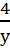### Algebraic Expressions 3

Mathematics J.S.S 2 Second Term

Theme: Algebraic Process

Sub Theme: Algebraic Operation

WEEK 3 & 4

Algebraic Expressions 3

Performance Objectives

Students should be able to;

1. Simplify algebraic expression on fractions with monomial denominators.
2. Interpret word problems involving algebraic fractions
3. Solve word problems involving algebraic fractions.

Algebraic Fractions with Monomial Denominators

Content

Algebraic fractions are simply fractions with algebraic expressions on the top and/or bottom.

Note; When adding or subtracting algebraic fractions, the first thing to do is to put them onto a common denominator (by cross multiplying).

Algebraic expressions are said to have monomial denominator if the denominator has a single term. Examples are xy, 4a, b2, etc. However, algebraic fraction with monomial denominators is of the form: